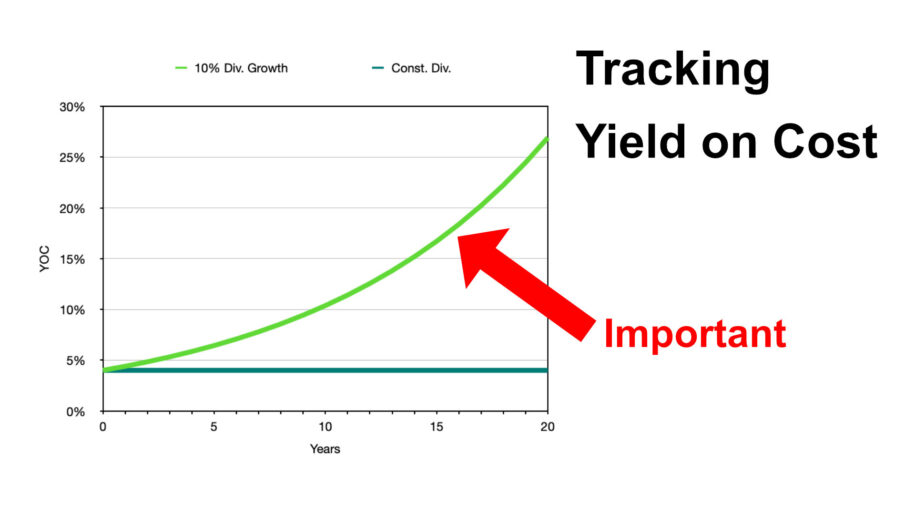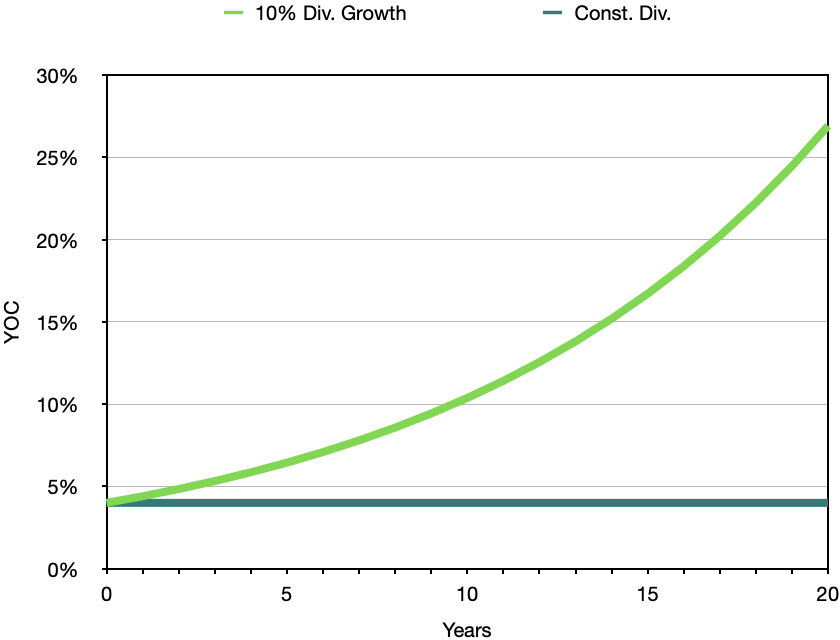# Do you track your Yield on Cost?Yield on Cost (YOC) is a metric that measures the annual dividend income received from an investment relative to the original cost of that investment. It’s calculated by dividing the annual dividend per share by the initial purchase price per share and then expressing it as a percentage:

YOC = (Annual Dividend per Share / Initial Purchase Price per Share) x 100

### Example

Imagine you bought shares of a company for \$100 each, and it pays an annual dividend of \$4 per share.

So, your initial YOC would be:

YOC = (\$4 / \$100) x 100 = 4.00%

## Changing Share Price

Now, imagine the share price rises to \$150 after your purchase.

How does this affect the YOC?

YOC = (\$4 / \$100) x 100 = 4.00%

It doesn’t, since the YOC calculation only considers the initial purchase price and the current dividend yield.

However, it does change the capital appreciation:

Capital Appreciation = (\$150 – \$100) / \$100 = 50%

The capital gain in this case would be 50%.

## Dividend Growth

The true power of dividend investing lies in dividend growth.

The YOC showcases the potential for exponential growth in dividend income over time.

Let’s illustrate this with a hypothetical example:

As the company continues to grow and succeed, it may increase its dividend payout per share over the years. Let’s say the company decides to raise its dividend by 10% each year.

After one year, the new annual dividend would be:

Year 1 Dividend per Share = \$4 + (\$4 x 10%) = \$4.40

Now, let’s calculate the new YOC after one year with the updated dividend:

Year 1 YOC = (\$4.40 / \$100) x 100 = 4.4%

Notice that the YOC has increased without doing anything. You were just holding your shares for one year.

Over time, as the company keeps raising its dividends each year, your YOC will continue to grow. After ten years, assuming the 10% dividend increase per year, your YOC would be around 10%. After 20 years, it would be about 27%.This graph compares two cases: (a) a yearly dividend increase by 10%, with (b) a constant dividend of 4%.

This means that even if the company’s dividend yield stays constant (the percentage of dividends relative to the current stock price), your YOC will continue to rise due to the original lower purchase price. This is the power of exponential growth in dividend income through Yield on Cost.

## Tracking Your Yield on Cost

As a dividend investor, you want to track the YOC of each of your investments.

How do you track the YOC of your stocks?

Calculating the YOC of a stock position manually in Excel is tedious since you have to consider all buy and sell transactions.

If you want you can do it as an exercise to get a better understanding. However, you will realize that it is a task you want to be done automatically.

The 22 Dividends app does this calculation for you. You just enter your buy and sell transactions and the application uses the stock’s dividend history to compute it.

The YOC value is visualized for each of your stock positions on the Portfolio screen.

The 22 Dividend app depicts the following values:

• Mkt. Yield: The current dividend YOC if you buy shares right now.
• Avg. Start Yield: The average start YOC of all of your transactions.
• YOC: The current YOC of your stock positions considering all buy and sell transactions, as well as the dividend history.

Tracking the YOC is really important since humans often underestimate the effects of exponential growth over time.